# 一行Python代码能实现什么丧心病狂的功能？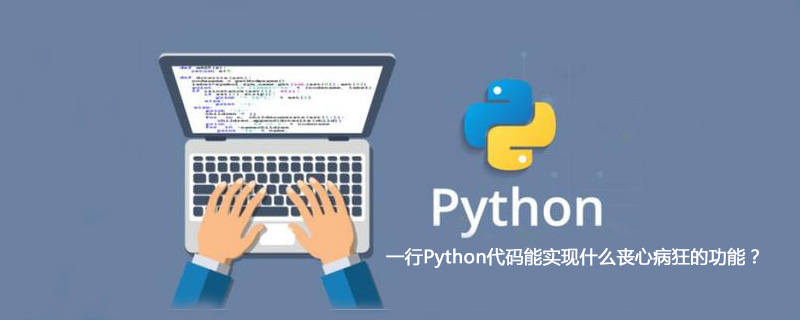1、 爱心

```print'\n'.join([''.join([(u'ILoveChina爱'[(x-y)%11]if((x*0.05)**2+(y*0.1)**2-1)**3-(x*0.05)**2*(y*0.1)**3<=0 else' ')
for x in range(-30,30)])for y in range(15,-15,-1)])```2、 美丽的螺旋

`exec """\nfrom turtle import *\nfor i in range(500): \n forward(i)\n left(91)\n"""`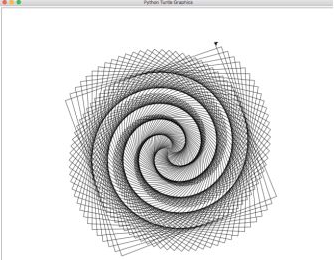1、猜数字

```python3 -c "import random;n=random.randint(1,99);[(lambda a:print('Y' if a==n else 'H' if a>n else 'L'))
(int(input())) for i in range(6)]”```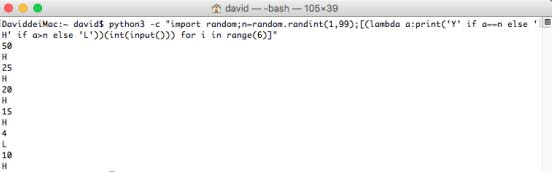2、老虎机

```python3 -c "import random;p=lambda:random.choice('7♪♫♣♠♦♥◄☼☽');[print('|'.join([p(),p(),p()]),end='\r')
for i in range(8**5)]”```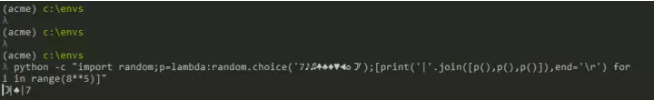3、 一行代码帮你选彩票号码

`python -c "from random import sample; print 'Lotto numbers: %s' % sample(range(1, 50), 6)"`1、一行代码实现GUI用户界面

`python -c "from Tkinter import *;root = Tk();w = Label(root, text='你好！慕课网！');w.pack();root.mainloop()”`2、一行代码启动一个http服务器

`python3 -m http.server 8888`3、Python的哲学

`python -c "import this"`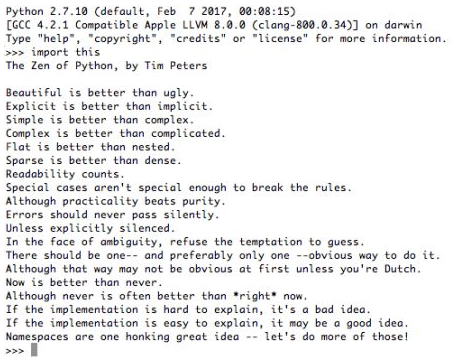4、 简易计算器

`print(eval(input()))`

5、 今天距离明年元旦还有多少天？

```python -c """import datetime;today = datetime.date.today();someday = datetime.date(2019, 1, 1);diff = someday
- today;print str(diff.days)+' days' “""```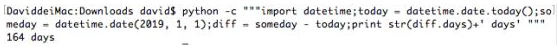1、换脸术

`print("#"+''.join(["{:0>2}".format(hex(int(a))[2:])for a in __import__('sys').argv[1:]]))`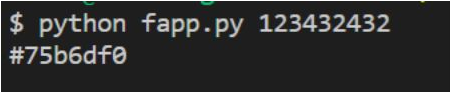2、星罗阵

`[print('\n'.join(" "*abs(c)+"* "*(a-abs(c))for c in range(-a+1,a))) for a in[int(__import__('sys').argv)]]`3、操控术

`[y.run() for y in [(x, x.route('/')(lambda :'Hello, world!')) for x in [__import__('flask').Flask(__name__)]]]`

4、凌波微步

```[plt.show() for _, __, ___, plt in [(plt.plot(x, y1, color='r'), plt.plot(x, y2, color='r'), np, plt) for x, y1, y2,
np, plt in [(x, 0.618*np.abs(x) - 0.8* np.sqrt(64-x**2), 0.618*np.abs(x) + 0.8* np.sqrt(64-x**2), np, plt) for x, np,
plt in [(np.linspace(-8, 8, 1024), np, plt) for np, plt in [(__import__('numpy'), __import__('pylab'))]]]]]```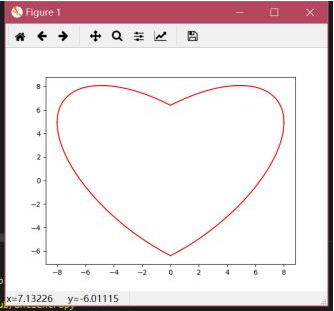5、吸星大法

```[print(decode(model.predict(x))) for model, x, decode in [(ResNet50(weights='imagenet'), preprocess_input
for ResNet50, image, preprocess_input, np, decode in [(kav.applications.resnet50.ResNet50, kp.preprocessing.image,
kav.applications.resnet50.preprocess_input, np, kav.applications.resnet50.decode_predictions) for kav, kp, np in
[(__import__('keras.applications.resnet50'), __import__('keras.preprocessing.image'), __import__('numpy'))]]]]```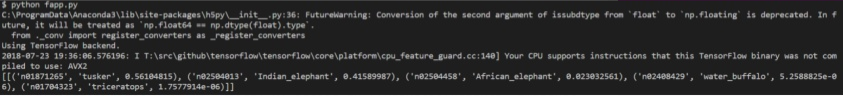Python开发开发项目